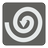Square Spiral
Simple
English JA RUNikola picks up a strange circuit board. All of its elements are connected in a spiral and it is possible to connect the neighboring elements vertically and horizontally.

The map of the circuit consists of a series of square cells. The first element in the center is marked as 1, and continuing in a clockwise spiral, each other elements is marked in ascending order. On the map, you can move (connect cells) vertically and horizontally. You can help Nikola find the manhattan distance between any two elements on the map. For example, the distance between cells 1 and 9 is two moves and the distance between 24 and 9 is one move.Input: Two marks of cells as an integers.

Output: The manhattan distance between the two cells as an integer.

Example:

```find_distance(1, 9) == 2
find_distance(9, 1) == 2
find_distance(10, 25) == 1
find_distance(5, 9) == 4

```

How it is used: This task will help you examine the non-standard coordinate systems. Sometime this model of the coordinates is using in the topography. And this task is related with the Ulam spiral (the prime Spiral). The Ulam spiral is a simple method of visualizing the prime numbers that reveals the apparent tendency of certain quadratic polynomials to generate unusually large numbers of primes

Precondition: 0 < first < 1000
0 < second < 1000
first != second